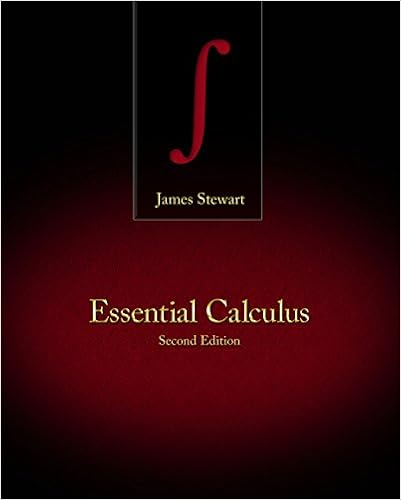# Activity 10 given the graph to a system of

• Notes
• 97
• 100% (11) 11 out of 11 people found this document helpful

This preview shows page 61 - 64 out of 97 pages.

##### We have textbook solutions for you!
The document you are viewing contains questions related to this textbook.The document you are viewing contains questions related to this textbook.
Chapter 11 / Exercise 9
Essential Calculus
StewartExpert Verified
Activity 10: Given the graph to a system of inequalities, the student will list three points that are solutions to the system, to each inequality, and to none of the inequalities.Algebra IUnit 5Systems of Equations and Inequalities58
##### We have textbook solutions for you!
The document you are viewing contains questions related to this textbook.The document you are viewing contains questions related to this textbook.
Chapter 11 / Exercise 9
Essential Calculus
StewartExpert Verified
Louisiana Comprehensive Curriculum, Revised 2008Algebra 1Unit 6: MeasurementTime Frame: Approximately three weeksUnit DescriptionThis unit is an advanced study of measurement. It includes the topics of precision and accuracy and investigates the relationship between the two. The investigation of absolute and relative error and how they each relate to measurement is included. Significant digits are also studied as well as how computations on measurements are affected when considering precision and significant digits.Student UnderstandingsStudents should be able to find the precision of an instrument and determine the accuracyof a given measurement. They should know the difference between precision and accuracy. Students should see error as the uncertainty approximated by an interval aroundthe true measurement. They should be able to calculate and use significant digits to solve problems.Guiding Questions1.Can students determine the precision of a given measurement instrument?2.Can students determine the accuracy of a measurement?3.Can students differentiate between what it means to be precise and what it means to be accurate?4.Can students discuss the nature of precision and accuracy in measurement andnote the differences in final measurement values that may result from error?5.Can students calculate using significant digits?Unit 6 Grade-Level Expectations (GLEs)GLE #GLE Text and BenchmarksNumber and Number Relations4.Distinguish between an exact and an approximate answer, and recognize errors introduced by the use of approximate numbers with technology (N-3-H) (N-4-H) (N-7-H)5.Demonstrate computational fluency with all rational numbers (e.g., estimation, mental math, technology, paper/pencil) (N-5-H)Algebra IUnit 6MeasurementPage 59
Louisiana Comprehensive Curriculum, Revised 2008Measurement17.Distinguish between precision and accuracy (M-1-H)GLE #GLE Text and Benchmarks18.Demonstrate and explain how the scale of a measuring instrument determines the precision of that instrument (M-1-H)19.Use significant digits in computational problems (M-1-H) (N-2-H)20.Demonstrate and explain how relative measurement error is compounded when determining absolute error (M-1-H) (M-2-H) (M-3-H)21.Determine appropriate units and scales to use when solving measurement problems (M-2-H) (M-3-H) (M-1-H)Sample ActivitiesActivity 1: What Does it Mean to be Accurate? (GLEs: 4, 17)Materials List: paper, pencil, three or more different types of scales from science department, three or more different bathroom scales, student’s watches, Internetaccess, What Does It Mean To Be Accurate? BLM, sticky notesThis unit on measurement will have many new terms to which students have not yet been exposed. Have students maintain a vocabulary self-awareness chart(view literacy strategy descriptions
•••